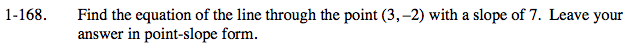### Home > CALC > Chapter 1 > Lesson 1.4.3 > Problem1-168

1-168.

Find the equation of the line through the point (3, −2) with a slope of 7. Leave your answer in point-slope form. Homework Help ✎The equation for point-slope form is y = m(xx1) + y1.